# Asg 2: Basic image convolution and edge detection

## Assignment

Implement the basic image convolution and, then use the (serabale) Sobel operators (a.k.a. kernels), to perform edge detection.

## Suggestions (e.g., for C implementation)

1. This assignment relies on convolution of a Finite Impulse Response (FIR) filter over the image. You could do this with a small 2D array, but, as an exercise, it convenient to follow this a 1D filtering approach where 2 1D separable filters are used.
2. The Sobel edge detection operators are likely the easiest to implement.

## Further Suggestions

1. For debugging a good idea is to write out and look at the processed image itself–because of the range of values, however, the output image may look strange. Typically normalizing the (float) values to range $$[0, 1]$$ prior to output is what is required.
2. The main() funcrtion can look something like this (with two filters, dx and dy for vertical and horizontal edges, e.g., see this page for more info): int main(int argc,char **argv) { PGM *gimg=NULL; PGM *ndximg=NULL, *ndyimg=NULL; PGM *dximg=NULL, *dyimg=NULL; FLTR *gxp=make_filter(0), *gyp=make_filter(1); // allocte image of given size gimg = pgm_read("mandrill.pgm"); // convolve dximg = pgm_conv(gimg,gxp); dyimg = pgm_conv(gimg,gyp); // copy edge image and normalize, for visualization purposes ndximg = pgm_copy(dximg); pgm_normalize(ndximg); pgm_write(ndximg,"ndxgrey.pgm"); ndyimg = pgm_copy(dyimg); pgm_normalize(ndyimg); pgm_write(ndyimg,"ndygrey.pgm"); // output to file pgm_write(dximg,"dxgrey.pgm"); pgm_write(dyimg,"dygrey.pgm"); // free image by sending pointer to pointer to ppm_free function pgm_free(&dximg); pgm_free(&dyimg); pgm_free(&gimg); free_filter(&gxp); free_filter(&gyp); return 0; } 

## Example Program Input & Output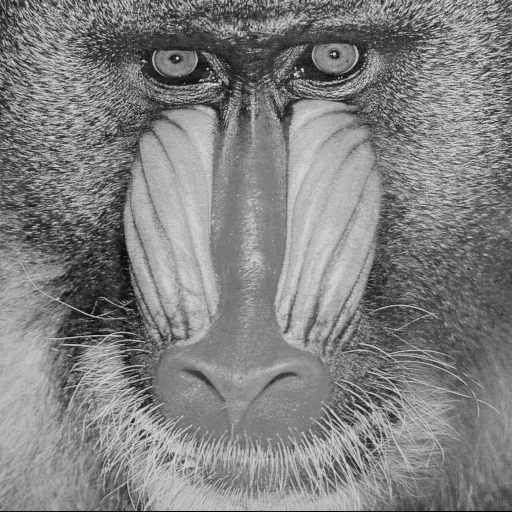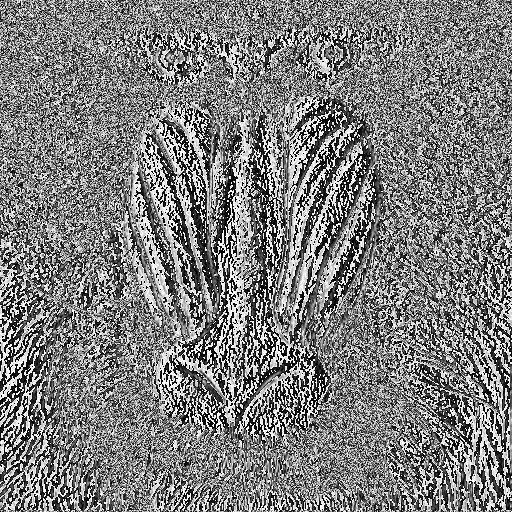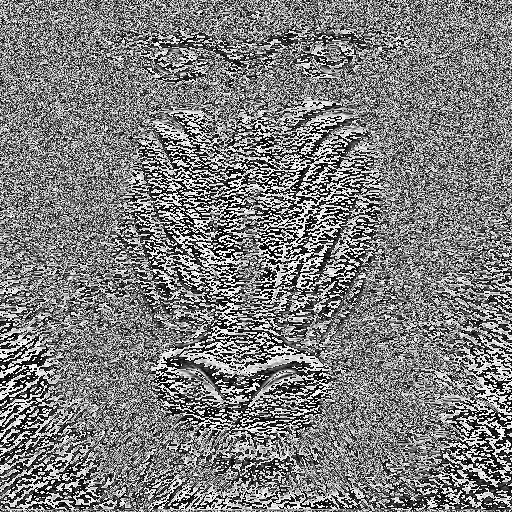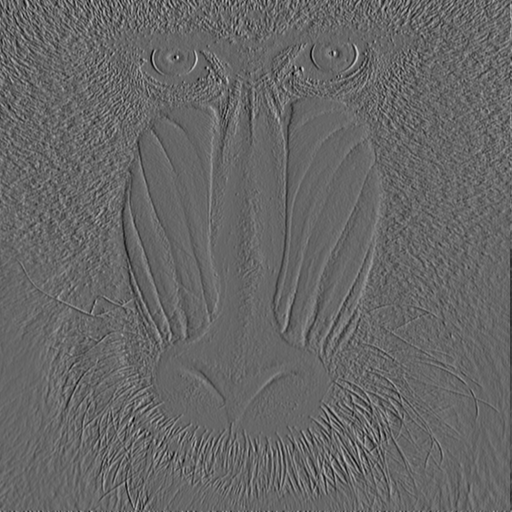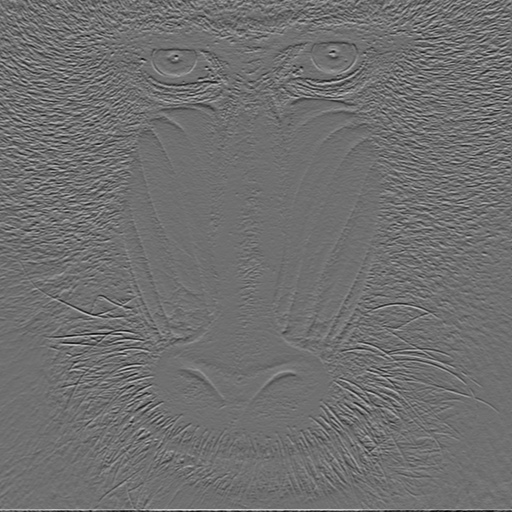## Supplemental

1. If you call your source code file main.c here is a Makefile that you can use to try to compile the project: CC = gcc INCLUDE = -I. CFLAGS = -g LDFLAGS = -L. -L/usr/lib LDLIBS = -lc -lm .c.o: $(CC)$(INCLUDE) $(CFLAGS) -c -o$@ $< OBJECTS = \ pgm.o \ arrays.o \ filters.o all: main main: main.o main.c$(CC) $(CFLAGS)$(INCLUDE) -o $@$@.o $(OBJECTS)$(LDFLAGS) \$(LDLIBS) main.o: main.c clean: rm -f *.o rm -rf main  Note that each line underneath the targets is indented by a tab, not just spaces, this is important!

## Turn in

Turn in all of your code, in one tar.gz archive of your asg##/ directory, including:
1. A README file containing
1. Course id--section no
2. Name
3. Brief solution description (e.g., program design, description of algorithm, etc., however appropriate).
4. Lessons learned, identified interesting features of your program
5. Any special usage instructions
2. Makefile
3. source code (.h headers and .c source)
4. object code (do a make clean before tar)

## How to hand in

See handin notes